# Analyzing Mobile Data Speeds from TRAI with Pandas

Python is a great language for doing data analysis, primarily because of the fantastic ecosystem of data-centric Python packages. Pandas is one of those packages and makes importing and analyzing data much easier.

Let’s use a real dataset from TRAI to analyze mobile dataspeeds and try to see the average speeds for a particular operator or state in that month. This will also show how easily Pandas could be used on any real world data to derive interesting results.

Telecom Regulatory Authority of India (TRAI) releases a monthly dataset of the internet speeds it measures through the MySpeed (TRAI) app. This includes speed tests initiated by the user itself or periodic background tests done by the app. We will try to analyze this dataset and see the average speeds for a particular operator or state in that month.

Inspecting the raw structure of data: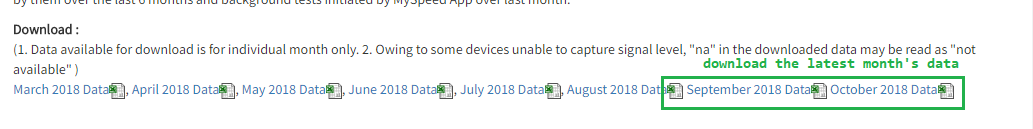NOTE: As the dataset is huge, the software may give you an warning that all rows could not be loaded. This is fine. Also if you are using Microsoft Excel, there might be a warning about opening of a SYLK file. This error could be ignored as it is a common bug in Excel.
Now, let’s take a look at the arrangement of the data-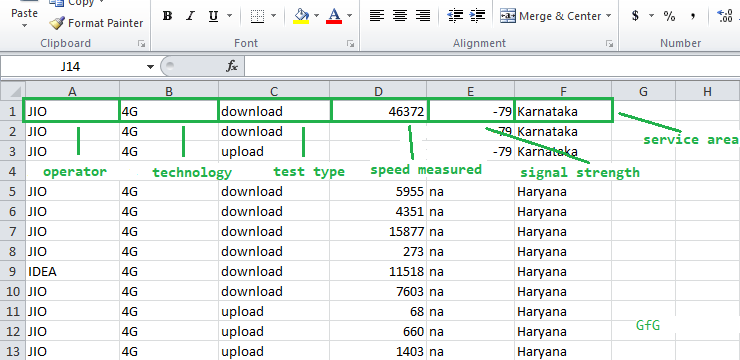Column Names in the dataset

1st column is of the Network OperatorJIO, Airtel etc.
2nd column is of the Network Technology3G or 4G.
4th column is the Speed Measured in Kilobytes per second.
5th column is the Signal Strength during the measurement.
6th column is the Local Service Area(LSA), or the circle where the test was done – Delhi, Orissa etc. We will refer to this as simply ‘states’.

• NOTE: The Signal Strength may have `na (Not Available)` values due to some devices unable to capture signal. We will ignore using this parameter in our calculations to make things simpler. However, it could be easily added as a condition while filtering.

Packages required –

Pandas – a popular data analysis toolkit. Very powerful for crunching large sets of data.
Numpy – provides fast and efficient operations on arrays of homogeneous data. We will use this to along with pandas and matplotlib.
Matplotlib – is a plotting library. We will use its bar plotting function to make bar graphs.

Lets start analyzing the data.

Step #1: Import the packages and define some constants.

 `import` `pandas as pd ` `import` `numpy as np ` `import` `matplotlib.pyplot as plt ` ` `  `# we will define some constants ` ` `  `# name of the csv dataset ` `DATASET_FILENAME ``=` `'sept18_publish.csv'` ` `  `# define the operator to be filtered upon. ` `CONST_OPERATOR ``=` `'JIO'` ` `  `# define the state to be filtered upon. ` `CONST_STATE ``=` `'Delhi'` ` `  `# define the the technology to be filtered upon ` `CONST_TECHNOLOGY ``=` `'4G'`

Step #2: Define some lists that will store the final calculated results, so that it could be passed on to the bar plotting function easily. The state (or operator), download speed and upload speed will be stored serially so that for an index, the state (or operator), it’s corresponding download and upload speeds can be accessed.

For Example, `final_states, final_download_speeds` and `final_upload_speeds` will give the corresponding values for the 3rd state.

 `# define lists ` `final_download_speeds ``=` `[] ` `final_upload_speeds ``=` `[] ` `final_states ``=` `[] ` `final_operators ``=` `[] `

Step #3: Import the file using Pandas `read_csv()` function and store it in ‘df’. This will create a DataFrame of the csv contents on which we will work on.

 `df ``=` `pd.read_csv(DATASET_FILENAME) ` ` `  `# assign headers for each of the columns based on the data ` `# this allows us to access columns easily ` ` `  `df.columns ``=` `[``'Service Provider'``, ``'Technology'``, ``'Test Type'``, ` `                   ``'Data Speed'``, ``'Signal Strength'``, ``'State'``] `

Step #4: First lets find all the unique states and operators in this dataset and store them into corresponding states and operators list.

We will use the `unique()` method of the Pandas dataframe.

 `# find and display the unique states ` `states ``=` `df[``'State'``].unique() ` `print``(``'STATES Found: '``, states) ` ` `  `# find and display the unique operators ` `operators ``=` `df[``'Service Provider'``].unique() ` `print``(``'OPERATORS Found: '``, operators) `

Output:

```STATES Found:  ['Kerala' 'Rajasthan' 'Maharashtra' 'UP East' 'Karnataka' nan
'North East' 'Mumbai' 'Chennai' 'Himachal Pradesh' 'Jammu & Kashmir'
'West Bengal']
OPERATORS Found:  ['IDEA' 'JIO' 'AIRTEL' 'VODAFONE' 'CELLONE']```

Step #5: Define the function `fixed_operator`, which will keep the operator constant and iterate through all the available states for that operator. We can construct a similar function for a fixed state.

 `# filter out the operator and technology ` `# first as this will be common for all ` `filtered ``=` `df[(df[``'Service Provider'``] ``=``=` `CONST_OPERATOR)  ` `               ``& (df[``'Technology'``] ``=``=` `CONST_TECHNOLOGY)] ` ` `  `# iterate through each of the states ` `for` `state ``in` `states: ` ` `  `    ``# create new dataframe which contains ` `    ``# only the data of the current state ` `    ``base ``=` `filtered[filtered[``'State'``] ``=``=` `state] ` ` `  `    ``# filter only download speeds based on test type ` `    ``down ``=` `base[base[``'Test Type'``] ``=``=` `'download'``] ` ` `  `    ``# filter only upload speeds based on test type ` `    ``up ``=` `base[base[``'Test Type'``] ``=``=` `'upload'``] ` ` `  `    ``# calculate mean of speeds in Data Speed ` `    ``# column using the Pandas.mean() method ` `    ``avg_down ``=` `down[``'Data Speed'``].mean() ` ` `  `    ``# calculate mean of speeds ` `    ``# in Data Speed column ` `    ``avg_up ``=` `up[``'Data Speed'``].mean() ` ` `  `    ``# discard values if mean is not a number(nan) ` `    ``# and append only the valid ones ` `    ``if` `(pd.isnull(avg_down) ``or` `pd.isnull(avg_up)): ` `        ``down, up ``=` `0``, ``0` `     `  `    ``else``: ` `        ``final_states.append(state) ` `        ``final_download_speeds.append(avg_down) ` `        ``final_upload_speeds.append(avg_up) ` ` `  `        ``# print output upto 2 decimal places ` `        ``print``(``str``(state) ``+` `' -- Avg. Download: '` `+` `                          ``str``(``'%.2f'` `%` `avg_down) ``+`  `         ``'  Avg. Upload: '` `+` `str``(``'%.2f'` `%` `avg_up)) `

Output:

```Kerala -- Avg. Download: 26129.27  Avg. Upload: 5193.46

### Plotting the data –

Use the `arange()` method of Numpy which returns evenly spaced values within a given interval. Here, passing the length of the `final_states` list, hence we get values from 0 to the number of states in the list like [0, 1, 2, 3 …]
We can then use these indices to plot a bar at that location. The second bar is plotted by offsetting the location of the first bar by the bar width.

 `fig, ax ``=` `plt.subplots() ` `  `  `# the width of each bar ` `bar_width ``=` `0.25` `  `  `# opacity of each bar ` `opacity ``=` `0.8` `  `  `# store the positions ` `index ``=` `np.arange(``len``(final_states)) ` `  `  `# the plt.bar() takes in the position ` `# of the bars, data to be plotted, ` `# width of each bar and some other  ` `# optional parameters, like the opacity and colour ` `  `  `# plot the download bars ` `bar_download ``=` `plt.bar(index, final_download_speeds, ` `                       ``bar_width, alpha``=``opacity, ` `                       ``color``=``'b'``, label``=``'Download'``) ` `# plot the upload bars   ` `bar_upload ``=` `plt.bar(index ``+` `bar_width, final_upload_speeds,  ` `                        ``bar_width, alpha``=``opacity, color``=``'g'``, ` `                                             ``label``=``'Upload'``) ` `  `  `# title of the graph ` `plt.title(``'Avg. Download/Upload speed for '` `                     ``+` `str``(CONST_OPERATOR)) ` `  `  `# the x-axis label ` `plt.xlabel(``'States'``) ` `  `  `# the y-axis label ` `plt.ylabel(``'Average Speeds in Kbps'``) ` `  `  `# the label below each of the bars, ` `# corresponding to the states ` `plt.xticks(index ``+` `bar_width, final_states, rotation``=``90``) ` `  `  `# draw the legend ` `plt.legend() ` `  `  `# make the graph layout tight ` `plt.tight_layout() ` `  `  `# show the graph ` `plt.show() `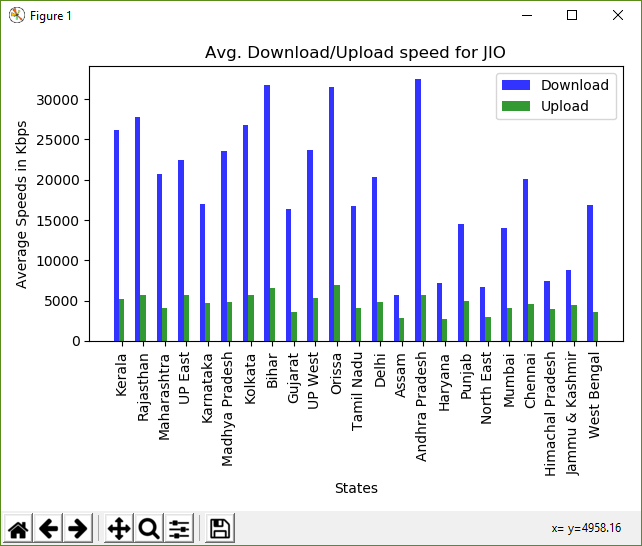Bar plot of the calculated speeds

### Comparing data of two months –

Let’s take some another month’s data as well and plot them together to observe the difference in the data speeds.

For this example, the previous month’s dataset will be same sept18_publish.csv and the next month’s dataset is oct18_publish.csv.

We just need to execute the same steps again. Read the another month’s data. Filter it out to subsequent dataframes and then plot it using a slightly different method. During plotting of the bars, we will increment the 3rd and 4th bars(corresponding to the second file’s upload and download) by 2 and 3 times the bar width, so that they are in their correct positions.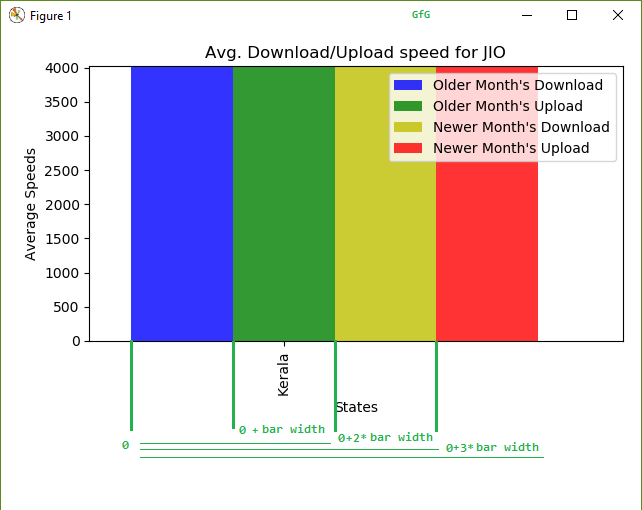Offsetting logic when plotting 4 bars

Below is the implementation for comparing 2-months of data:

 `import` `pandas as pd ` `import` `numpy as np ` `import` `matplotlib.pyplot as plt ` `import` `time ` `  `  `# older month ` `DATASET_FILENAME ``=` `'https://myspeed.trai.gov.in/download/sept18_publish.csv'` `  `  `# newer month ` `DATASET_FILENAME2 ``=` `'https://myspeed.trai.gov.in/download/oct18_publish.csv'` `  `  `CONST_OPERATOR ``=` `'JIO'` `CONST_STATE ``=` `'Delhi'` `CONST_TECHNOLOGY ``=` `'4G'` `  `  `# read file with Pandas and store as Dataframe ` `df ``=` `pd.read_csv(DATASET_FILENAME) ` `df2 ``=` `pd.read_csv(DATASET_FILENAME2) ` `  `  `# assign column names ` `df.columns ``=` `[``'Service Provider'``, ``'Technology'``, ``'Test Type'``, ` `                   ``'Data Speed'``, ``'Signal Strength'``, ``'State'``] ` `df2.columns ``=` `[``'Service Provider'``, ``'Technology'``, ``'Test Type'``, ` `                    ``'Data Speed'``, ``'Signal Strength'``, ``'State'``] ` `  `  `# find and display the unique states ` `states ``=` `df[``'State'``].unique() ` `print``(``'STATES Found: '``, states) ` `  `  `# find and display the unique operators ` `operators ``=` `df[``'Service Provider'``].unique() ` `print``(``'OPERATORS Found: '``, operators) ` `  `  `# define lists ` `final_download_speeds ``=` `[] ` `final_upload_speeds ``=` `[] ` `  `  `final_download_speeds_second ``=``[] ` `final_upload_speeds_second ``=` `[] ` `      `  `final_states ``=` `[] ` `final_operators ``=` `[] ` `  `  `# assign column names to the data ` `df.columns ``=` `[``'Service Provider'``, ``'Technology'``, ``'Test Type'``,  ` `                   ``'Data Speed'``, ``'Signal Strength'``, ``'State'``] ` `df2.columns ``=` `[``'Service Provider'``, ``'Technology'``, ``'Test Type'``, ` `                    ``'Data Speed'``, ``'Signal Strength'``, ``'State'``] ` `  `  ` `  `print``(``'\n\nComparing data for'` `+` `str``(CONST_OPERATOR)) ` `filtered ``=` `df[(df[``'Service Provider'``] ``=``=` `CONST_OPERATOR) ` `               ``& (df[``'Technology'``] ``=``=` `CONST_TECHNOLOGY)] ` ` `  `filtered2 ``=` `df2[(df2[``'Service Provider'``] ``=``=` `CONST_OPERATOR) ` `                 ``& (df2[``'Technology'``] ``=``=` `CONST_TECHNOLOGY)] ` `  `  `for` `state ``in` `states: ` `    ``base ``=` `filtered[filtered[``'State'``] ``=``=` `state] ` ` `  `    ``# calculate mean of download speeds ` `    ``avg_down ``=` `base[base[``'Test Type'``] ``=``=` `               ``'download'``][``'Data Speed'``].mean() ` ` `  `    ``# calculate mean of upload speeds ` `    ``avg_up ``=` `base[base[``'Test Type'``] ``=``=` `                 ``'upload'``][``'Data Speed'``].mean() ` ` `  `    ``base2 ``=` `filtered2[filtered2[``'State'``] ``=``=` `state] ` ` `  `    ``# calculate mean of download speeds ` `    ``avg_down2 ``=` `base2[base2[``'Test Type'``] ``=``=`  `             ``'download'``][``'Data Speed'``].mean() ` ` `  `    ``# calculate mean of upload speeds ` `    ``avg_up2 ``=` `base2[base2[``'Test Type'``] ``=``=`  `                ``'upload'``][``'Data Speed'``].mean() ` ` `  ` `  `    ``# discard values if mean is not a number(nan) ` `    ``# and append only the needed speeds ` `    ``if` `(pd.isnull(avg_down) ``or` `pd.isnull(avg_up) ``or` `        ``pd.isnull(avg_down2) ``or` `pd.isnull(avg_up2)): ` `        ``avg_down ``=` `0` `        ``avg_up ``=` `0` `        ``avg_down2 ``=` `0` `        ``avg_up2 ``=` `0` `    ``else``: ` `        ``final_states.append(state) ` `        ``final_download_speeds.append(avg_down) ` `        ``final_upload_speeds.append(avg_up) ` `        ``final_download_speeds_second.append(avg_down2) ` `        ``final_upload_speeds_second.append(avg_up2) ` `         `  `        ``print``(``'Older: '` `+` `str``(state) ``+` `' -- Download: '` `+` `              ``str``(``'%.2f'` `%` `avg_down) ``+` `'  Upload: '` `+` `              ``str``(``'%.2f'` `%` `avg_up)) ` ` `  `        ``print``(``'Newer: '` `+` `str``(state) ``+` `' -- Download: '` `+` `              ``str``(``'%.2f'` `%` `avg_down2) ``+` `'  Upload: '` `+` `              ``str``(``'%.2f'` `%` `avg_up2)) ` ` `  `# plot bargraph ` `fig, ax ``=` `plt.subplots() ` `index ``=` `np.arange(``len``(final_states)) ` `bar_width ``=` `0.2` `opacity ``=` `0.8` ` `  `rects1 ``=` `plt.bar(index, final_download_speeds,  ` `                 ``bar_width, alpha``=``opacity, color``=``'b'``, ` `                     ``label``=``'Older Month\'s Download'``) ` ` `  `rects2 ``=` `plt.bar(index ``+` `bar_width, final_upload_speeds,  ` `                    ``bar_width, alpha``=``opacity, color``=``'g'``, ` `                          ``label``=``'Older Month\'s Upload'``) ` ` `  `rects3 ``=` `plt.bar(index ``+` `2` `*` `bar_width, final_download_speeds_second,  ` `                                 ``bar_width, alpha``=``opacity, color``=``'y'``, ` `                                     ``label``=``'Newer Month\'s Download'``) ` ` `  `rects4 ``=` `plt.bar(index ``+` `3` `*` `bar_width, final_upload_speeds_second,  ` `                               ``bar_width, alpha``=``opacity, color``=``'r'``, ` `                                     ``label``=``'Newer Month\'s Upload'``) ` ` `  `plt.xlabel(``'States'``) ` `plt.ylabel(``'Average Speeds'``) ` `plt.title(``'Avg. Download/Upload speed for '` `                     ``+` `str``(CONST_OPERATOR)) ` ` `  `plt.xticks(index ``+` `bar_width, final_states, rotation``=``90``) ` `plt.legend() ` `plt.tight_layout() ` ` `  `plt.show() `

Output:

```STATES Found:  ['Kerala' 'Rajasthan' 'Maharashtra' 'UP East' 'Karnataka' nan
'North East' 'Mumbai' 'Chennai' 'Himachal Pradesh' 'Jammu & Kashmir'
'West Bengal']
OPERATORS Found:  ['IDEA' 'JIO' 'AIRTEL' 'VODAFONE' 'CELLONE']```
```Comparing data forJIO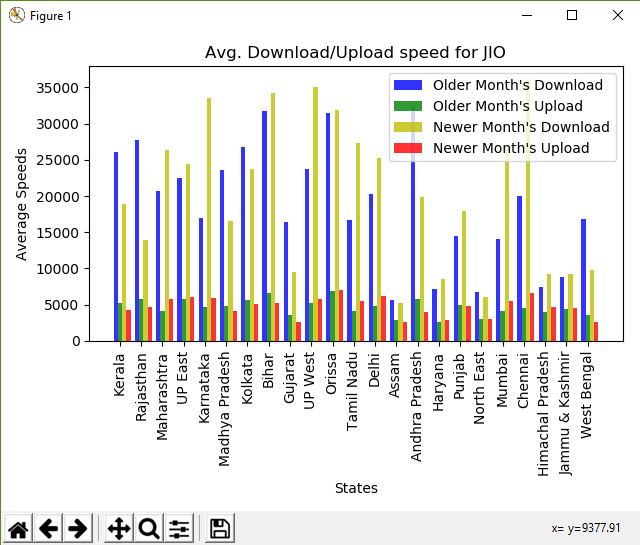Bar plot of the output

We just learned how to analyze some real world data and draw some interesting observations from it. But note that not all the data will be as nicely formatted and simple to deal with, Pandas makes it incredibly easy to work with such datasets.

My Personal Notes arrow_drop_upCheck out this Author's contributed articles.

If you like GeeksforGeeks and would like to contribute, you can also write an article using contribute.geeksforgeeks.org or mail your article to contribute@geeksforgeeks.org. See your article appearing on the GeeksforGeeks main page and help other Geeks.

Please Improve this article if you find anything incorrect by clicking on the "Improve Article" button below.

Article Tags :

79

Please write to us at contribute@geeksforgeeks.org to report any issue with the above content.